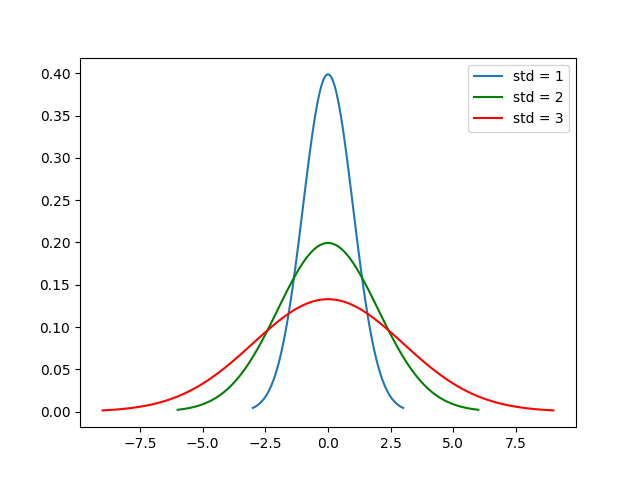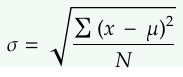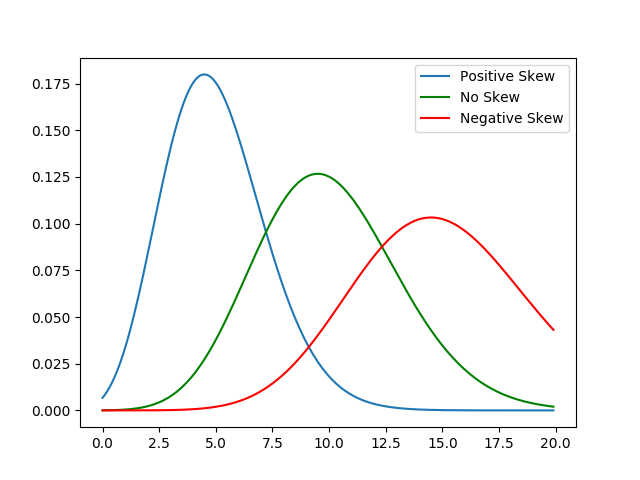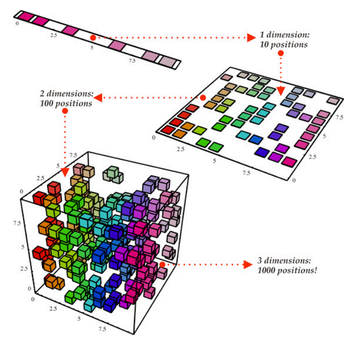#5 Useful Statistics Data Scientists Need to Know

A data scientist should know how to effectively use statistics to gain insights from data. Here are five useful and practical statistical concepts that every data scientist must know.commentsData Science can be practically defined as the process by which we get extra information from data. When doing Data Science, what we’re really trying to do is explain what all of the data actually means in the real-world, beyond the numbers.

To extract the information embedded in complex datasets, Data Scientists employ a number of tools and techniques including data exploration, visualisation, and modelling. One very important class of mathematical technique often used in data exploration is statistics.

In a practical sense, statistics allows us to define concrete mathematical summaries of our data. Rather than trying to describe every single data point, we can use statistics to describe some of its properties. And that’s often enough for us to extract some kind of information about the structure and make-up of the data.

Sometimes, when people hear the word “statistics” they think of something overly complicated. Yes, it can get a bit abstract, but we don’t always need to resort to the complex theories to get some kind of value out of statistical techniques.

The most basic parts of statistics can often be of the most practical use in Data Science.

Today, we’re going to look at 5 useful Statistics for Data Science. These won’t be crazy abstract concepts but rather simple, applicable techniques that go a long way.

Let’s get started!

### (1) Central Tendency

The central tendency of a dataset or feature variable is the center or typical value of the set. The idea is that there may be one single value that can best describe (to an extent) our dataset.

For example, imagine if you had a normal distribution centered at the x-y position of (100, 100). Then the point (100, 100) is the central tendency since, out of all the points to choose from, it is the one that provides the best summary of the data.

For Data Science, we can use central tendency measures to get a quick and simple idea of how our dataset looks as a whole. The “center” of our data can be a very valuable piece of information, telling us how exactly the dataset is biased, since whichever value the data revolves around is essentially a bias.

There are 2 common ways of mathematically selecting a central tendency.

Mean

The Mean value of a dataset is the average value i.e. a number around which a whole data is spread out. All values used in calculating the average are weighted equally when defining the Mean.

For example, let’s calculate the Mean of the following 5 numbers:

(3 + 64 + 187 + 12 + 52) / 5 = 63.6

The mean is great for computing the actual mathematical average. It’s also very fast to compute with Python libraries like Numpy

Median

Median is the middle value of the dataset i.e if we sort the data from smallest to biggest (or biggest to smallest) and then take the value in the middle of the set: that’s the Median.

Let’s again compute the Median for that same set of 5 numbers:

[3, 12, 52, 64, 187] → 52

The Median value is quite different from the Mean value of 63.6. Neither of them are right or wrong, but we can pick one based on our situation and goals.

Computing the Median requires sorting the data — this won’t be practical if your dataset is large.

On the other hand the Median will be more robust to outliers than the Mean, since the Mean will be pulled one way or the other if there are some very high magnitude outlier values.

The mean and median can be calculated with simple numpy one-liners:

```numpy.mean(array)
numpy.median(array)```

Under the umbrella of Statistics, the spread of the data is the extent to which it is squeezed towards a single value or more spread out across a wider range.

Take a look at the plots of the Gaussian probability distributions below — imagine that these are probability distributions describing an real-world dataset.

The blue curve has the smallest spread value since most of its data points all fall within a fairly narrow range. The red curve has the largest spread value since most of the data points take up a much wider range.

The legend shows the standard deviation values of these curves, explained in the next section.Standard Deviation

The standard deviation is the most common way of quantifying the spread of a data. Calculating it involves 5 steps:

1. Find the mean.
2. For each data point, find the square of its distance to the mean.
3. Sum the values from Step 2.
4. Divide by the number of data points.
5. Take the square root.A larger value means that our data is more “spread out” from the mean. A smaller value means that our data is more concentrated around the mean.

Easily calculate the standard deviation in Numpy like so:

`numpy.std(array)`

### (3) Percentiles

We can further describe the position of each data point throughout the range using percentiles.

The percentile describes the exact position of the data point in terms of how high or low it is positioned in the range of values.

More formally, the pth percentile is the value in the dataset at which it can be split into two parts. The lower part contains p percent of the data i.e the pth percentile.

For example, consider the set of 11 numbers below:

`1, 3, 5, 7, 9, 11, 13, 15, 17, 19, 21`

The number 15 is the 70th percentile since when we split the dataset into 2 parts at the number 15, 70% of the remaining data is less than 15.

Percentiles combined with the mean and standard deviation can give us a good idea of where a specific point lies within the spread / range of our data. If it’s an outlier, then its percentile will be close to the ends — less than 5% or great than 95%. On the other hand, if the percentile is calculated as close to 50 then we know that it’s close our our central tendency.

The 50th percentile for an array can be calculated in Numpy like so:

`numpy.percentile(array, 50)`

### (4) Skewness

The Skewness of data measures its asymmetry.

A positive value for the skewness means that values are concentrated on the left of the center of the data points; negative skewness means values are concentrated on the right of the center of the data points.

The graph below provides a nice illustration.We can calculate the skewness with the following equation:Skewness will give us an idea of how close our data’s distribution is to being Gaussian. The greater the magnitude of the skewness, the further from a Gaussian distribution our dataset is.

This is important because if we have a rough idea of our data’s distribution, we can tailor whatever ML model we are going to train for that particular distribution. Moreover, not all ML modelling techniques will be effective on data which is not Gaussian.

Once again, stats gives us insightful information before we jump right into modelling!

Here’s how we can compute the Skewness in Scipy code:

`scipy.stats.skew(array)`

### (5) Covariance and Correlation

Covariance

The covariance of two features variables measures how “related” they are. If the two variables have a positive covariance, then when one variable increases so does the other; with a negative covariance the values of the feature variables will change in opposite directions.

Correlation

Correlation is simply the normalised (scaled) covariance where we divide by the product of the standard deviation of the two variables being analysed. This effectively forces the range of correlation to always be between -1.0 and 1.0.

If the correlation of two feature variables is 1.0, then the variables have a perfect positive correlation. This means that if one variable changes by a given amount, the second moves proportionally in the same direction.Illustration of PCA used for Dimensionality Reduction

A positive correlation coefficient less than one indicates a less than perfect positive correlation, with the strength of the correlation growing as the number approaches one. The same idea works for negative values of correlation, just with the values of the feature variables changing in opposite directions rather than changing in the same direction.

Knowing about correlation is incredibly useful for techniques like Principal Component Analysis (PCA) used for Dimensionality Reduction. We start by computing a correlation matrix — if there are 2 or more variables which are highly correlated, then they are effectively redundant in explaining our data and some of them can be dropped to reduce the complexity.

Bio: George Seif is a Certified Nerd and AI / Machine Learning Engineer.

Related:

By subscribing you accept KDnuggets Privacy Policy

By subscribing you accept KDnuggets Privacy Policy

By subscribing you accept KDnuggets Privacy Policy